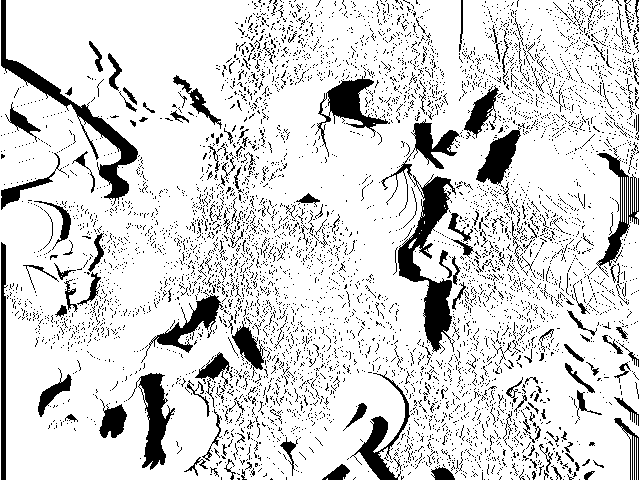# Smpling binary map from a grid (generating occlusion-meaning mask from depth warping)

Hello, I implemented depth warping just using inverse_warp.py from DPSNet for 480*640 image and its depth map

I want to create a binary map with the target coordinates of depth warping as 1 and the other coordinates as 0. In other words, it means displaying the occlusion-exposed background as 0 and the non-occlusion as 1. (What I want is not an occlusion mask, but it represents occlusion by marking the region that was occluded as 0.)

``````    src_pixel_coords = cam2pixel(cam_coords, proj_cam_to_src_pixel[:,:,:3], proj_cam_to_src_pixel[:,:,-1:], padding_mode)  # [B,H,W,2]

return projected_feat, src_pixel_coords
``````

To achieve this, I used invers_warp.py to return src_pixel_coords (i.e. target coordinates), which is passed as an argument to the torch.nn.functional.grid_sample() function.

From this, src_pixel_coords value, I can produce the binary map I desire by following numpy code:

``````warped_img, src_pixel_coords = inverse_warp( ... )

img_height = 480
img_width = 640

coords = (src_pixel_coords + 1) / 2       #0-1 normalize
x_coords = coords[0,:,:,0] * img_width  #batch_size = 1
y_coords = coords[0,:,:,1] * img_height

x_coords = x_coords.floor().clamp(0,img_width-1).cpu().numpy()
y_coords = y_coords.floor().clamp(0,img_height-1).cpu().numpy()

for y in range(img_height):
for x in range(img_width):
y_ = np.clip(2*y-int(y_coords[y,x]), 0, img_height-1)
x_ = np.clip(2*x-int(x_coords[y,x]), 0, img_width-1)